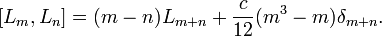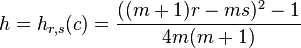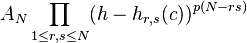# Black hole thermodynamics

In physics, black hole thermodynamics is the area of study that seeks to reconcile the laws of thermodynamics with the existence of black hole event horizons. Much as the study of the statistical mechanics of black body radiation led to the advent of the theory of quantum mechanics, the effort to understand the statistical mechanics of black holes has had a deep impact upon the understanding of quantum gravity, leading to the formulation of the holographic principle.

It is important that ones is able to see the progression from abstraction to a interpretation of foundational approach.

***

Andy Strominger:
This was a field theory that lived on a circle, which means it has one spatial dimension and one time dimension. We derived the fact that the quantum states of the black hole could be represented as the quantum states of this one-plus-one dimensional quantum field theory, and then we counted the states of this theory and found they exactly agreed with the Bekenstein-Hawking entropy.See:Quantum Microstates: Gas Molecules in the Presence of a Gravitational Field

See:Microscopic Origin of the Bekenstein-Hawking Entropy

Of course I am interested the mathematical framework as it might be compared to some phenomenological approach that gives substance to any theoretical thought.

For example, Tommaso Dorigo is a representative of the type of people who may affect the general distribution of "subjects" that may grow at CERN or the Fermilab in the next decade or two. And he just published a quote by Sherlock Holmes - no kidding - whose main point is that it is a "capital mistake" to work on any theory before the data are observed.See:Quantum gravity: minority report

I think you were a little harsh on Tommaso Dorigo  Lubos because he is really helping us to understand the scientific process at Cern. But you are right about theory in my mind, before the phenomenological approach can be seen. The mind need to play creatively in the abstract notions before it can be seen in it's correlations in reality.

***

# Virasoro algebra

Group theoryGroup theory
In mathematics, the Virasoro algebra (named after the physicist Miguel Angel Virasoro) is a complex Lie algebra, given as a central extension of the complex polynomial vector fields on the circle, and is widely used in string theory.

## Definition

The Virasoro algebra is spanned by elements
Li for$i\in\mathbf{Z}$
and c with
Ln + L n
and c being real elements. Here the central element c is the central charge. The algebra satisfies
[c,Ln] = 0
and$[L_m,L_n]=(m-n)L_{m+n}+\frac{c}{12}(m^3-m)\delta_{m+n}.$
The factor of 1/12 is merely a matter of convention.
The Virasoro algebra is a central extension of the (complex) Witt algebra of complex polynomial vector fields on the circle. The Lie algebra of real polynomial vector fields on the circle is a dense subalgebra of the Lie algebra of diffeomorphisms of the circle.
The Virasoro algebra is obeyed by the stress tensor in string theory, since it comprises the generators of the conformal group of the worldsheet, obeys the commutation relations of (two copies of) the Virasoro algebra. This is because the conformal group decomposes into separate diffeomorphisms of the forward and back lightcones. Diffeomorphism invariance of the worldsheet implies additionally that the stress tensor vanishes. This is known as the Virasoro constraint, and in the quantum theory, cannot be applied to all the states in the theory, but rather only on the physical states (confer Gupta-Bleuler quantization).

## Representation theory

A lowest weight representation of the Virasoro algebra is a representation generated by a vector v that is killed by Li for i ≥1 , and is an eigenvector of L0 and c. The letters h and c are usually used for the eigenvalues of L0 and c on v. (The same letter c is used for both the element c of the Virasoro algebra and its eigenvalue.) For every pair of complex numbers h and c there is a unique irreducible lowest weight representation with these eigenvalues.
A lowest weight representation is called unitary if it has a positive definite inner product such that the adjoint of Ln is Ln. The irreducible lowest weight representation with eigenvalues h and c is unitary if and only if either c≥1 and h≥0, or c is one of the values$c = 1-{6\over m(m+1)} = 0,\quad 1/2,\quad 7/10,\quad 4/5,\quad 6/7,\quad 25/28, \ldots$
for m = 2, 3, 4, .... and h is one of the values$h = h_{r,s}(c) = {((m+1)r-ms)^2-1 \over 4m(m+1)}$
for r = 1, 2, 3, ..., m−1 and s= 1, 2, 3, ..., r. Daniel Friedan, Zongan Qiu, and Stephen Shenker (1984) showed that these conditions are necessary, and Peter Goddard, Adrian Kent and David Olive (1986) used the coset construction or GKO construction (identifying unitary representations of the Virasoro algebra within tensor products of unitary representations of affine Kac-Moody algebras) to show that they are sufficient. The unitary irreducible lowest weight representations with c < 1 are called the discrete series representations of the Virasoro algebra. These are special cases of the representations with m = q/(pq), 0<r<q, 0< s<p for p and q coprime integers and r and s integers, called the minimal models and first studied in Belavin et al. (1984).
The first few discrete series representations are given by:
• m = 2: c = 0, h = 0. The trivial representation.
• m = 3: c = 1/2, h = 0, 1/16, 1/2. These 3 representations are related to the Ising model
• m = 4: c = 7/10. h = 0, 3/80, 1/10, 7/16, 3/5, 3/2. These 6 representations are related to the tri critical Ising model.
• m = 5: c = 4/5. There are 10 representations, which are related to the 3-state Potts model.
• m = 6: c = 6/7. There are 15 representations, which are related to the tri critical 3-state Potts model.
The lowest weight representations that are not irreducible can be read off from the Kac determinant formula, which states that the determinant of the invariant inner product on the degree h+N piece of the lowest weight module with eigenvalues c and h is given by$A_N\prod_{1\le r,s\le N}(h-h_{r,s}(c))^{p(N-rs)}$
which was stated by V. Kac (1978), (see also Kac and Raina 1987) and whose first published proof was given by Feigin and Fuks (1984). (The function p(N) is the partition function, and AN is some constant.) The reducible highest weight representations are the representations with h and c given in terms of m, c, and h by the formulas above, except that m is not restricted to be an integer ≥ 2 and may be any number other than 0 and 1, and r and s may be any positive integers. This result was used by Feigin and Fuks to find the characters of all irreducible lowest weight representations.

## Generalizations

There are two supersymmetric N=1 extensions of the Virasoro algebra, called the Neveu-Schwarz algebra and the Ramond algebra. Their theory is similar to that of the Virasoro algebra.
The Virasoro algebra is a central extension of the Lie algebra of meromorphic vector fields on a genus 0 Riemann surface that are holomorphic except at two fixed points. I.V. Krichever and S.P. Novikov (1987) found a central extension of the Lie algebra of meromorphic vector fields on a higher genus compact Riemann surface that are holomorphic except at two fixed points, and M. Schlichenmaier (1993) extended this to the case of more than two points.

## History

The Witt algebra (the Virasoro algebra without the central extension) was discovered by E. Cartan (1909). Its analogues over finite fields were studied by E. Witt in about the 1930s. The central extension of the Witt algebra that gives the Virasoro algebra was first found (in characteristic p>0) by R. E. Block (1966, page 381) and independently rediscovered (in characteristic 0) by I. M. Gelfand and D. B. Fuks (1968). Virasoro (1970) wrote down some operators generating the Virasoso algebra while studying dual resonance models, though he did not find the central extension. The central extension giving the Virasoro algebra was rediscovered in physics shortly after by J. H. Weis, according to Brower and Thorn (1971, footnote on page 167).

***